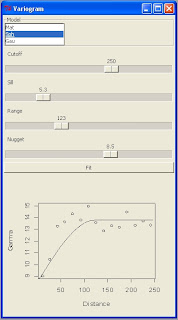## Wednesday, 21 September 2011

### Variogram fit with RPanel

During the UseR 2011 conference I saw lots of examples of the use of RPanel to create a GUI in R. Yesterday, because I was a bit bored of the work I was doing I started thinking about this and I decided to try this package.

My objective was to create a new panel with all the main setting for fitting a variogram model to an omnidirectional variogram with gstat.

This is the script:

library(rpanel)
library(gstat)

coordinates(data)=~Lat+Lon

##Variogram Fitting
variogram.plot <- function(panel) {
with(panel, {
variogram<-variogram(Oxigen~1,data,cutoff=Cutoff)
vgm.var<-vgm(psill=Sill,model=Model,range=Range,nugget=Nugget)
#fit<-fit.variogram(variogram,vgm.var)
plot(variogram\$dist,variogram\$gamma,xlab="Distance",ylab="Semivariance")
lines(variogramLine(vgm.var,maxdist=Range))
})
panel
}

var.panel <- rp.control("Variogram",Sill=20,Range=250,Nugget=0,Model="Mat",Cutoff=250)
rp.listbox(var.panel,Model,c("Mat","Sph","Gau"))
rp.slider(var.panel, Cutoff, 0,500,showvalue=T)
rp.slider(var.panel, Sill, 0,500,showvalue=T)
rp.slider(var.panel, Range, 0,1000,showvalue=T)
rp.slider(var.panel, Nugget, 0,15,showvalue=T)
rp.button(var.panel, title = "Fit", action = variogram.plot)

At this address you can find a zip file with a sample dataset that you can use to try this script, however if you know a bit of gstat you can start customizing it straigth away:

This is the screenshot from my R Console:I also tried to embed the variogram plot into the panel in order to execute the script in batch mode, this is the result:

if(print(require(tcltk))==FALSE){install.packages("tcltk",repos="http://cran.r-project.org")}
if(print(require(tcltk))==TRUE){require(tcltk)}

if(print(require(rpanel))==FALSE){install.packages("rpanel",repos="http://cran.r-project.org")}
if(print(require(rpanel))==TRUE){require(rpanel)}

if(print(require(gstat))==FALSE){install.packages("gstat",repos="http://cran.r-project.org")}
if(print(require(gstat))==TRUE){require(gstat)}

coordinates(data)=~Lat+Lon

grid <- spsample(data, cellsize = 10, type = "regular")
gridded(grid) <- TRUE

##Variogram Fitting
variogram.plot <- function(panel) {
with(panel, {
variogram<-variogram(Oxigen~1,data,cutoff=Cutoff)
vgm.var<-vgm(psill=Sill,model=Model,range=Range,nugget=Nugget)
#fit<-fit.variogram(variogram,vgm.var)
plot(variogram\$dist,variogram\$gamma,xlab="Distance",ylab="Gamma")
lines(variogramLine(vgm.var,maxdist=Range*2))
})
panel
}

replot.smooth <- function(object) {
rp.tkrreplot(var.panel, plot)
object
}

var.panel <- rp.control("Variogram",Sill=20,Range=250,Nugget=0,Model="Mat",Cutoff=250,size=c(800,600))
rp.listbox(var.panel,Model,c("Mat","Sph","Gau"))
rp.slider(var.panel, Cutoff, 0,sqrt(areaSpatialGrid(grid)),showvalue=T)
rp.slider(var.panel, Sill, 0,var(data\$Oxigen)*2,showvalue=T)
rp.slider(var.panel, Range, 0,sqrt(areaSpatialGrid(grid)),showvalue=T)
rp.slider(var.panel, Nugget, 0,var(data\$Oxigen),showvalue=T)
rp.button(var.panel, title = "Fit",action=replot.smooth)
rp.tkrplot(var.panel, plot,variogram.plot)
rp.block(var.panel)

It probably need some more work in order to be perfect but at the moment I'm not interested in a perfect automatic script.
If someone has time to spend on it and is able to made it perfectly automatic for every data file, please share the script with the community.

By the way, I also implemented a line from the tcltk package for the selection of the data file interactively.
This is the resulting panel:1.2.